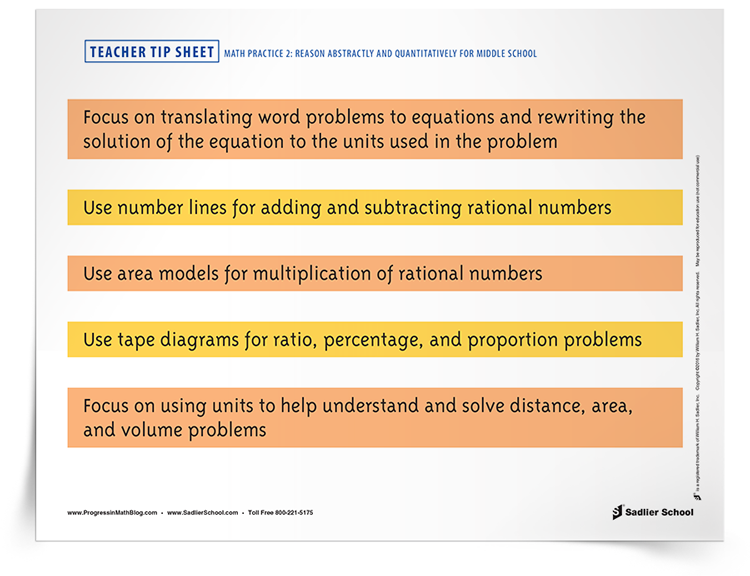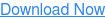1.800.221.5175
Core Program
Full Access
Core Program
Full Access
Core Program
Full Access
National Program
Full Access
New York
Critical Thinking for Active Math Minds
Preparing for Standards Based Assessments
Vocabulary Workshop, Tools for Comprehension Grades 1–5
Print Program
Interactive Edition
Print Program
Interactive Edition
Vocabulary Workshop, Tools for Excellence Grades 6–12+
Print Program
Interactive Edition
Vocabulary for Success
Progress English Language Arts Grades K–8
National Program
Full Access
New York
Grammar Workshop, Tools for Writing
Grammar for Writing
Writing a Research Paper
Writing Workshop
Print Program
Interactive Practice Bundle
Print Program
Interactive Edition

A K–8 resource to support deep comprehension of math skills and conceptsTopics:

#### May 12, 2016 k-2-math-practices, 6-8-math-practices, mp-abstract-quantitative, 3-5-math-practices

In this post I will share several ways that you can use Mathematical Practice 2 in your classroom. For me, this practice was one of the more confusing ones when I first tried to understand it. I hope that you will understand it better after I explain it to you. You will then be able to help develop your students’ abilities to reason abstractly and quantitatively. I am going to break the standard down into three parts. The download for this blog post is a tip sheet to remind you of these ideas.

##Part One: Translating word problems to equations — and vice versa — is one the most challenging areas for my students.

Mathematical Practice 2 gives us language that shows students the relationship between the word problem (quantitative) and the algebraic equation (abstract). Most standard word problems are about quantities, asking How many animals, how much money, how many degrees… The possibilities are endless.

When we take a word problem and translate it to an equation, we create an abstraction of the problem. For example, the equation x + 6 = 9 is an abstract problem. It could represent any of these situations:

1. I received six dollars from my cousin, and now I have nine dollars. How much money did I have when I started?

2. We went to the pet store and bought six more fish for our fish tank. We now have nine fish in the tank. How many fish did we have before we went to the store?

3. In six more years, I will be nine years old. How old am I now?

The quantities are dollars, fish, and age. The problems are quite different in that they are about different topics, but abstractly the problems can all be represented by the equation x + 6 = 9. We take word problems or real life situations and create equations that model the problems. This is one aspect of what it means to reason abstractly and quantitatively. The problem can be solved by subtracting six from both sides, giving the solution x = 3. Now we need to take this abstract idea and give it a quantity. For problem one, it would be \$3, the answer for problem two would be three fish, and problem three’s answer would be three years old. Our abstract solution (x = 3) becomes a real-life quantity (dollars, fish, years).

Sometimes students are so happy to have solved a problem that they forget to rewrite the solution as an answer to the question with a label. This step, attending to precision, is part of Mathematical Practice 2, as well as of Mathematical Practice 7.

## Part 2: Using the number line is another way that students can reason abstractly and quantitatively.

By middle school, students should be used to the number line for plotting numbers, for drawing lengths to represent numbers, and for showing addition, subtraction, multiplication and division. Modeling these quantities and operations as line segments, with arrows to show direction, results in abstractions of using the numbers and operations. These skills will become increasingly valuable as students expand the types of numbers they use to include integers and rational numbers (including negative fractions and decimals).

A specific skill that helps students with their number sense and with Math Practice 2 is to show them how to add and subtract rational numbers on the number line. First, by using a number line and drawing lines to approximate the size of the number, they can estimate the value of the answer. By fine tuning their estimates they are able to find the exact value of the answer. Using a two-number line system (or area model) to teach multiplying rational numbers is an extension of this idea.

As students progress to eighth grade and begin to develop their knowledge of irrational numbers, the number line will be even more important as they learn to approximate the value of irrational numbers (such as square roots) and to estimate the value of their sums and differences.

Similar to using the number line, using tape diagrams for ratio, percentage, and proportion problems is useful for teaching students how to reason abstractly and quantitatively.

## Part 3: When solving measurement problems, use units to help reasoning about the problem and to substitute values in known formulas.

For instance, in a word problem, 12 square centimeters refers to an area while 12 cubic centimeters refers to a volume, and 12 centimeters would refer to the side length, height, radius, diameter, circumference, or perimeter of a figure.

In middle school problems such as Find the height of a triangle with area of 24 square centimeters and a base of 12 centimeters requires students not just to calculate an area, but also to solve an equation where one of the linear dimensions is missing.

As part of Mathematical Practice 2, this use of reasoning about units of measurement leads directly to one of the high school number sense and quantity standards: Use units as a way to understand problems and to guide the solution of multi-step problems; choose and interpret units consistently in formulas; choose and interpret the scale and the origin in graphs and data displays.

To help your students reason abstractly and quantitatively in middle school you need to think about how to highlight these three areas in your teaching. For a reminder for you and your students about Mathematical Practice 2, click on the link below to print out a tip sheet.